# Index

of a numbermoduloThe exponentin the congruence, whereandare relatively prime integers andis a fixed primitive root modulo. The index ofmodulois denoted by, orfor short. Primitive roots exist only for moduli of the form, where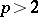is a prime number; consequently, the notion of an index is only defined for these moduli.

Ifis a primitive root moduloandruns through the values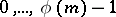, where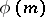is the Euler function, thenruns through a reduced system of residues modulo. Consequently, for each number relatively prime withthere exist a unique indexfor which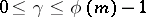. Any other index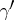ofsatisfies the congruence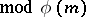. Therefore, the indices ofform a residue class modulo.

The notion of an index is analogous to that of a logarithm of a number, and the index satisfies a number of properties of the logarithm, namely: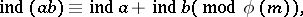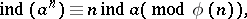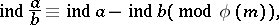where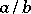denotes the root of the equation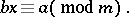If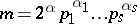is the canonical factorization of an arbitrary natural numberand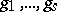are primitive roots modulo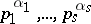, respectively, then for eachrelatively primitive withthere exist integers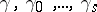for which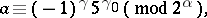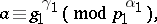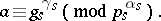The above systemis called a system of indices ofmodulo. To each numberrelatively prime withcorresponds a unique system of indicesfor which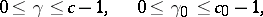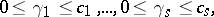where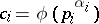,, andand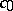and defined as follows:Every other systemof indices ofsatisfies the congruences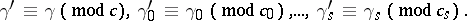The notion of a system of indices ofmodulois convenient for the explicit construction of characters of the multiplicative group of reduced residue classes modulo.